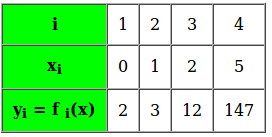# Lagrange’s Interpolation

What is Interpolation?
Interpolation is a method of finding new data points within the range of a discrete set of known data points (Source Wiki). In other words interpolation is the technique to estimate the value of a mathematical function, for any intermediate value of the independent variable.
For example, in the given table we’re given 4 set of discrete data points, for an unknown function f(x) :How to find?
Here we can apply the Lagrange’s interpolation formula to get our solution.
The Lagrange’s Interpolation formula:
If, y = f(x) takes the values y0, y1, … , yn corresponding to x = x0, x1 , … , xn then,This method is preferred over its counterparts like Newton’s method because it is applicable even for unequally spaced values of x.

We can use interpolation techniques to find an intermediate data point say at x = 3.

 `// C++ program for implementation of Lagrange's Interpolation ` `#include ` `using` `namespace` `std; ` ` `  `// To represent a data point corresponding to x and y = f(x) ` `struct` `Data ` `{ ` `    ``int` `x, y; ` `}; ` ` `  `// function to interpolate the given data points using Lagrange's formula ` `// xi corresponds to the new data point whose value is to be obtained ` `// n represents the number of known data points ` `double` `interpolate(Data f[], ``int` `xi, ``int` `n) ` `{ ` `    ``double` `result = 0; ``// Initialize result ` ` `  `    ``for` `(``int` `i=0; i

Output:

`Value of f(3) is : 35`

Complexity:
The time complexity of the above solution is O(n2) and auxiliary space is O(1).

References:
https://en.wikipedia.org/wiki/Lagrange_polynomial
Higher Engineering Mathematics , Dr. B.S. Grewal
https://mat.iitm.ac.in/home/sryedida/public_html/caimna/interpolation/lagrange.html

My Personal Notes arrow_drop_up

Article Tags :
Practice Tags :

2

Please write to us at contribute@geeksforgeeks.org to report any issue with the above content.